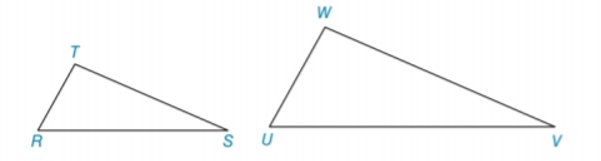Chapter 5.3, Problem 7EElementary Geometry For College St...

7th Edition
Alexander + 2 others
ISBN: 9781337614085

Solutions

Chapter
SectionElementary Geometry For College St...

7th Edition
Alexander + 2 others
ISBN: 9781337614085
Textbook Problem

In Exercises 5 to 8, name the method ( A A , S S S ~ ,   o r   S A S ~ ) that is used to show that the triangles are similar. ∠ T ≅ ∠ W and T R W U = T S W VTo determine

To name:

The method that is used to show that the triangles are similar.

Explanation

Given:

The triangles,

TW and TRWU=TSWV.

Approach:

Two polygons are similar if and only if two conditions are satisfied.

1. All pairs of corresponding angles are congruent.

2. All pairs of corresponding sides are proportional.

Definitions:

AA:

If the two angles of one triangle are congruent to the two angles of a another triangle, then the triangles are similar.

SAS:

If an angle of one triangle is congruent to an angle of a second triangle and the pairs of sides including the angles are proportional (in length), then the triangles are similar.

SSS:

If the three sides of one triangle are proportional (in length) to the three corresponding sides of a second triangle, then the triangles are similar

Still sussing out bartleby?

Check out a sample textbook solution.

See a sample solution

The Solution to Your Study Problems

Bartleby provides explanations to thousands of textbook problems written by our experts, many with advanced degrees!

Get Started

Simplify: 1236

Elementary Technical Mathematics

Evaluate the integral by making the given substitution. xex2dx,u=x2

Single Variable Calculus: Early Transcendentals, Volume I

Subtract 1125625.

Contemporary Mathematics for Business & Consumers

Find the critical numbers of the function. f(x) = x2 ln x

Single Variable Calculus: Early Transcendentals

Using n = 4 and midpoints for xi, then Riemann sum for 17x2 dx is a) 3443 b) 72 c) 168 d) 112

Study Guide for Stewart's Single Variable Calculus: Early Transcendentals, 8th

The range of is: (−∞,∞) [0, ∞) (0, ∞) [1, ∞]

Study Guide for Stewart's Multivariable Calculus, 8th